“堆”（Heap）这种数据结构的应用场景非常多，最经典的莫过于堆排序了。堆排序是一种原地的、时间复杂度为 $O(nlog⁡n)$ 的排序算法。

## 如何理解“堆”？

• 堆是一个完全二叉树；
• 堆中每一个节点的值都必须大于等于（或小于等于）其子树中每个节点的值。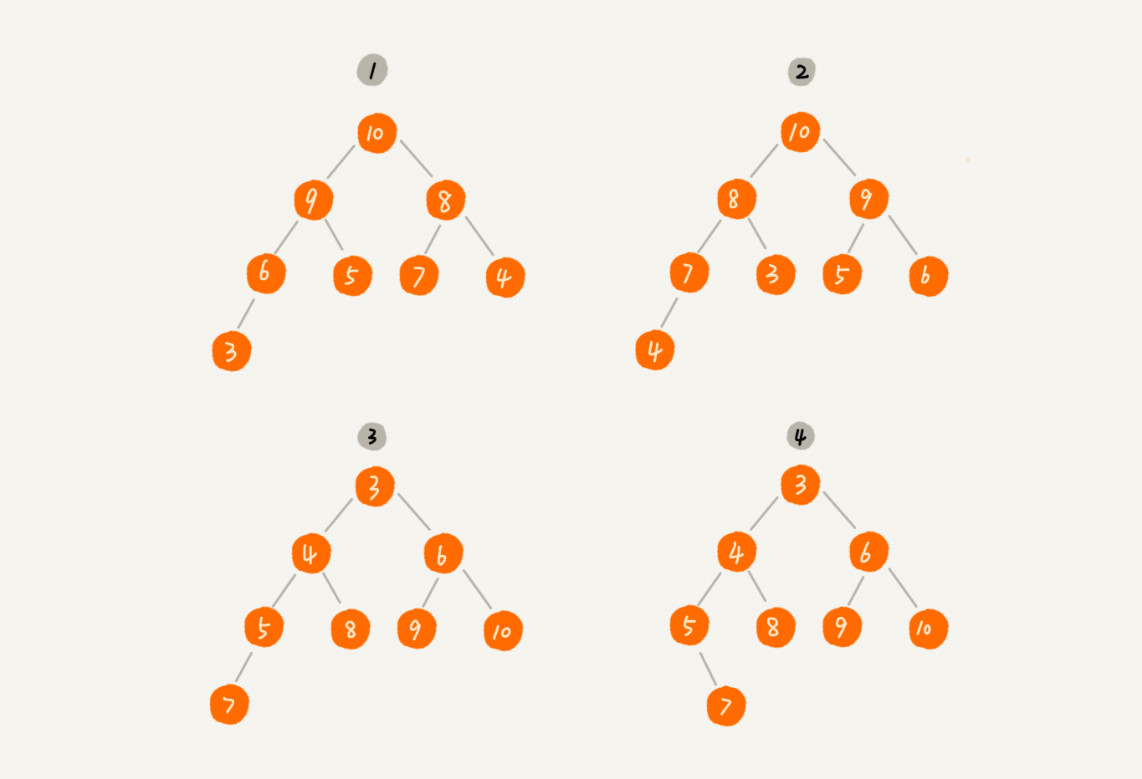## 如何实现一个堆？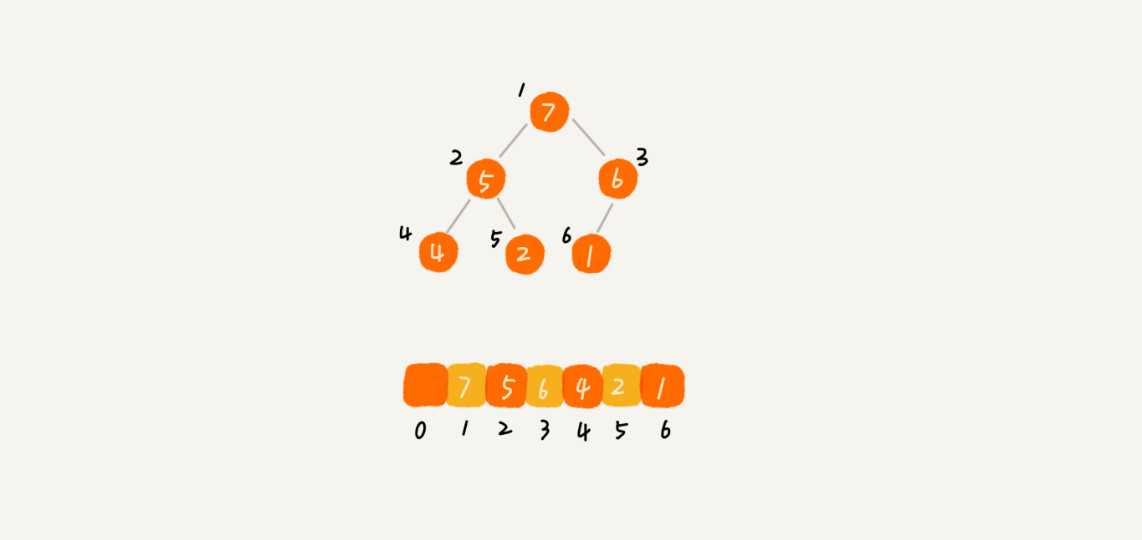1. 往堆中插入一个元素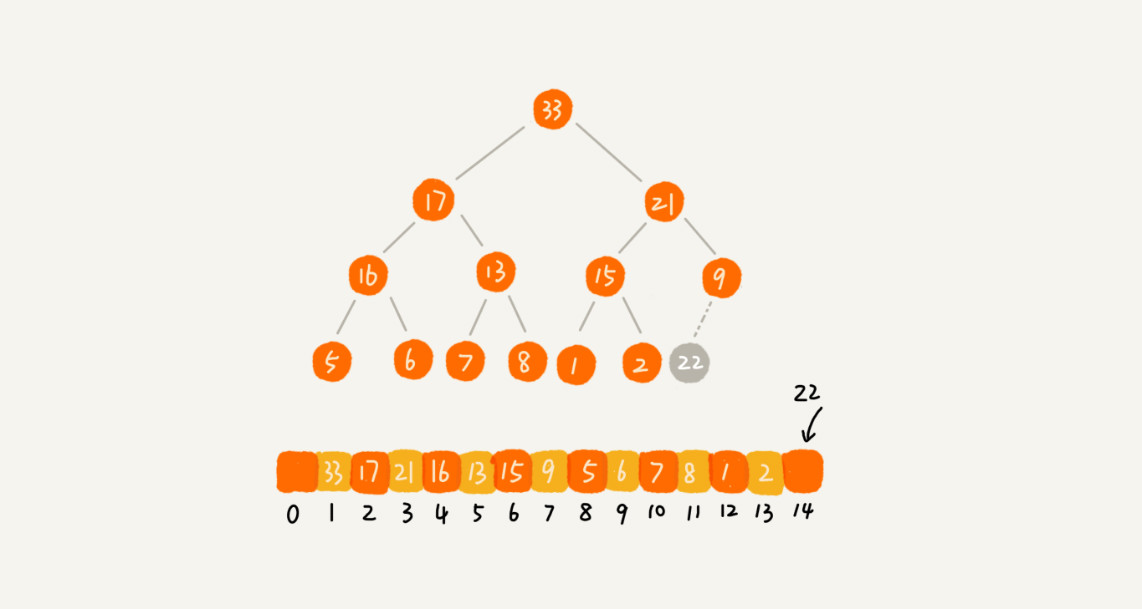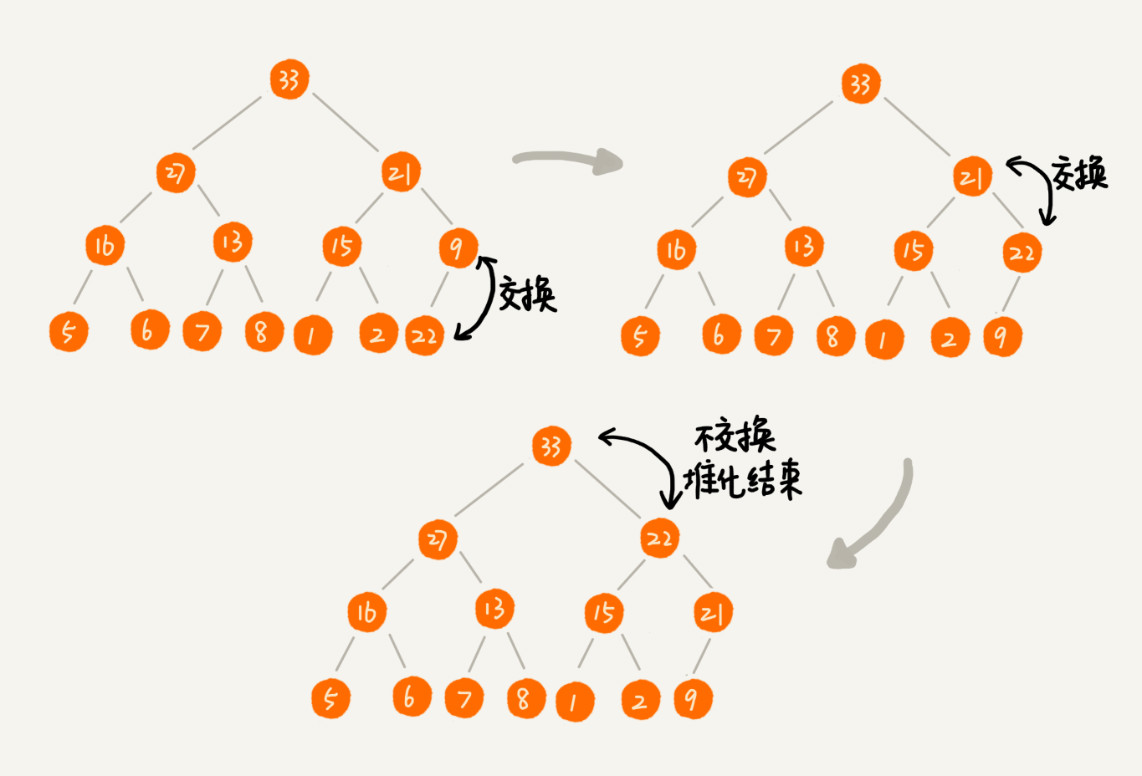public class Heap {
private int[] a; // 数组，从下标 1 开始存储数据
private int n;  // 堆可以存储的最大数据个数
private int count; // 堆中已经存储的数据个数

public Heap(int capacity) {
a = new int[capacity + 1];
n = capacity;
count = 0;
}

public void insert(int data) {
if (count >= n) return; // 堆满了
++count;
a[count] = data;
int i = count;
while (i/2 > 0 && a[i] > a[i/2]) { // 自下往上堆化
swap(a, i, i/2); // swap() 函数作用：交换下标为 i 和 i/2 的两个元素
i = i/2;
}
}
}

2. 删除堆顶元素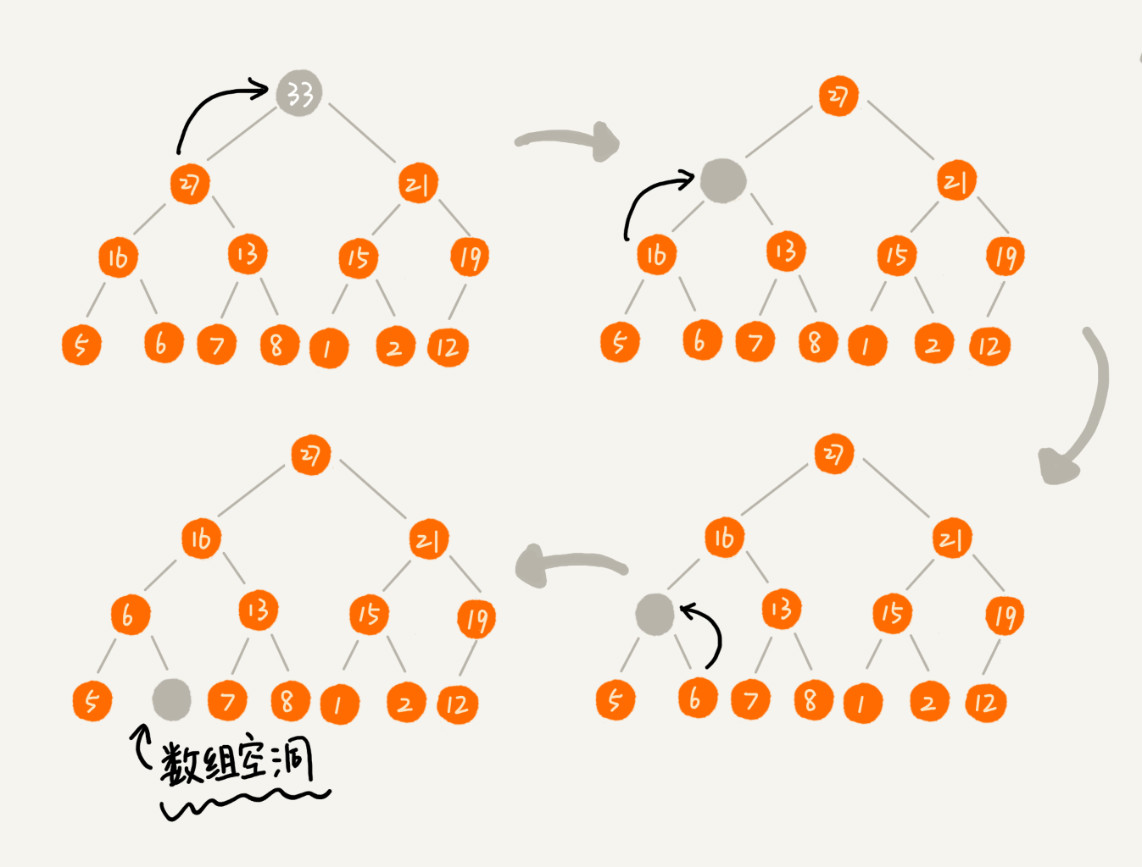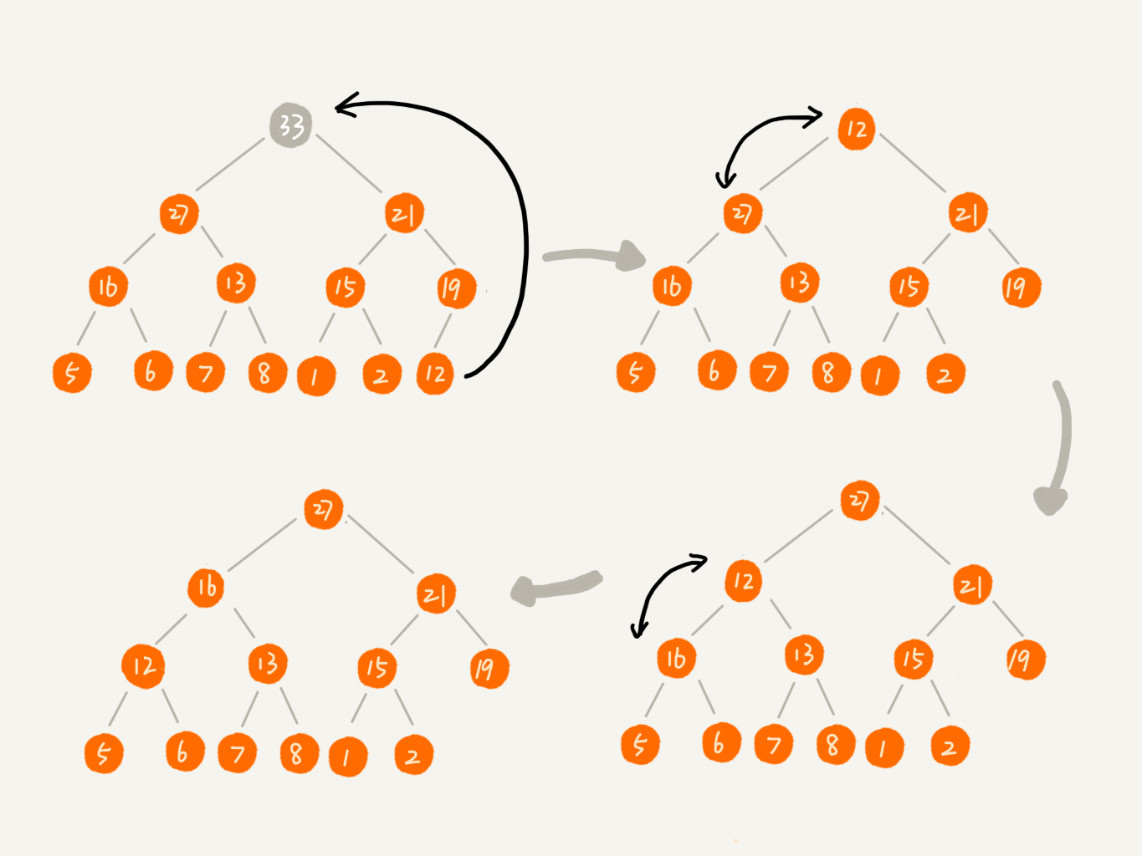public void removeMax() {
if (count == 0) return -1; // 堆中没有数据
a = a[count];
--count;
heapify(a, count, 1);
}

private void heapify(int[] a, int n, int i) { // 自上往下堆化
while (true) {
int maxPos = i;
if (i*2 <= n && a[i] < a[i*2]) maxPos = i*2;
if (i*2+1 <= n && a[maxPos] < a[i*2+1]) maxPos = i*2+1;
if (maxPos == i) break;
swap(a, i, maxPos);
i = maxPos;
}
}

## 如何基于堆实现排序？

1. 建堆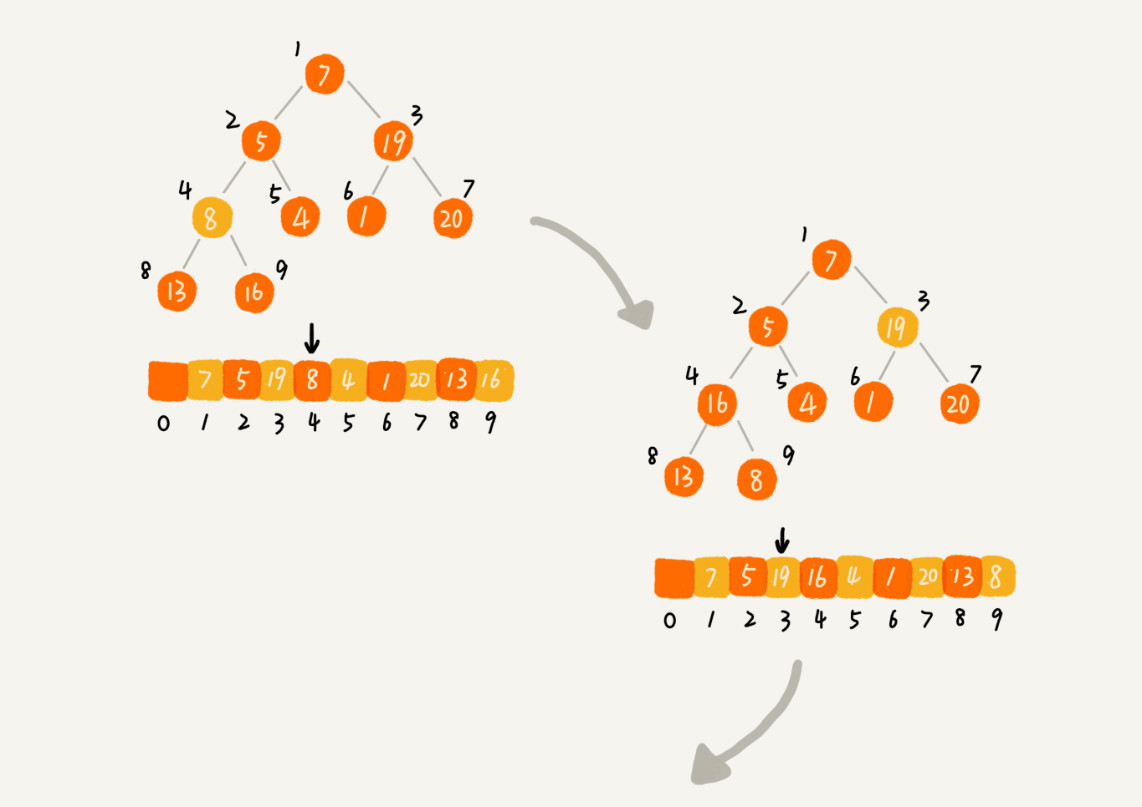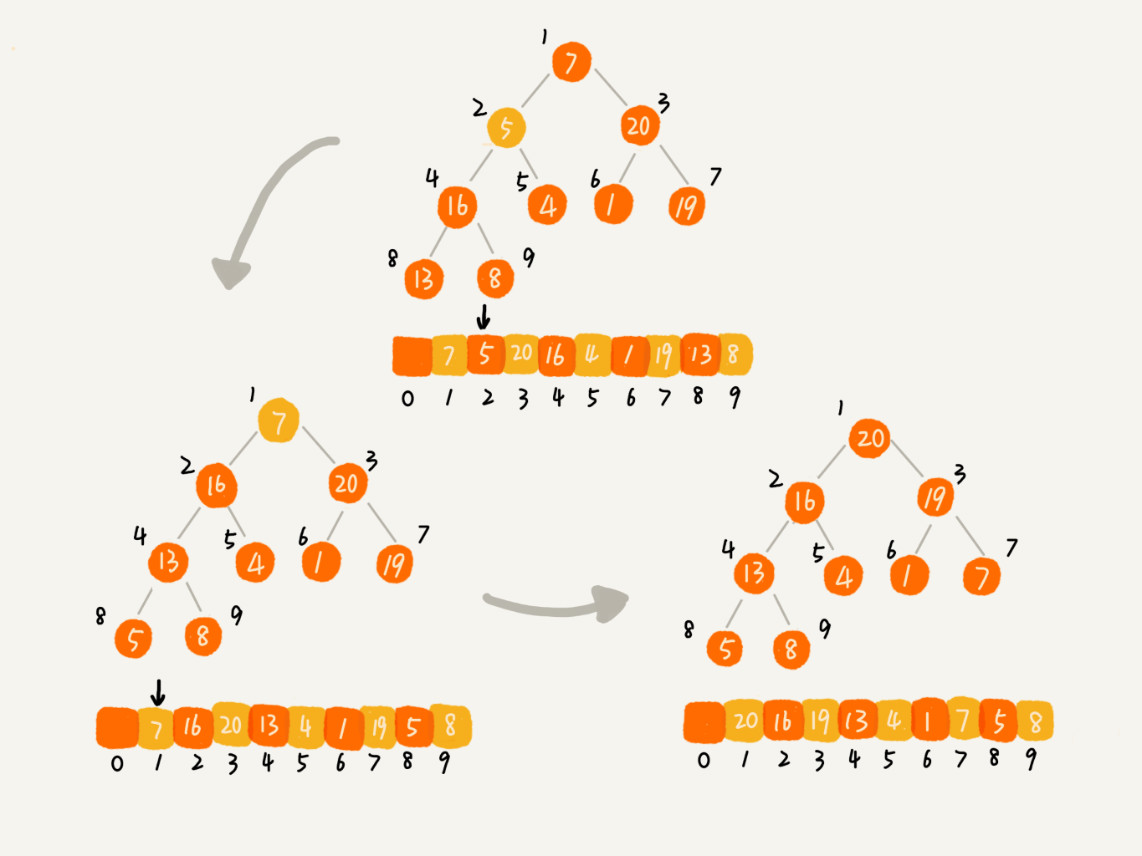private static void buildHeap(int[] a, int n) {
for (int i = n/2; i >= 1; --i) {
heapify(a, n, i);
}
}

private static void heapify(int[] a, int n, int i) {
while (true) {
int maxPos = i;
if (i*2 <= n && a[i] < a[i*2]) maxPos = i*2;
if (i*2+1 <= n && a[maxPos] < a[i*2+1]) maxPos = i*2+1;
if (maxPos == i) break;
swap(a, i, maxPos);
i = maxPos;
}
}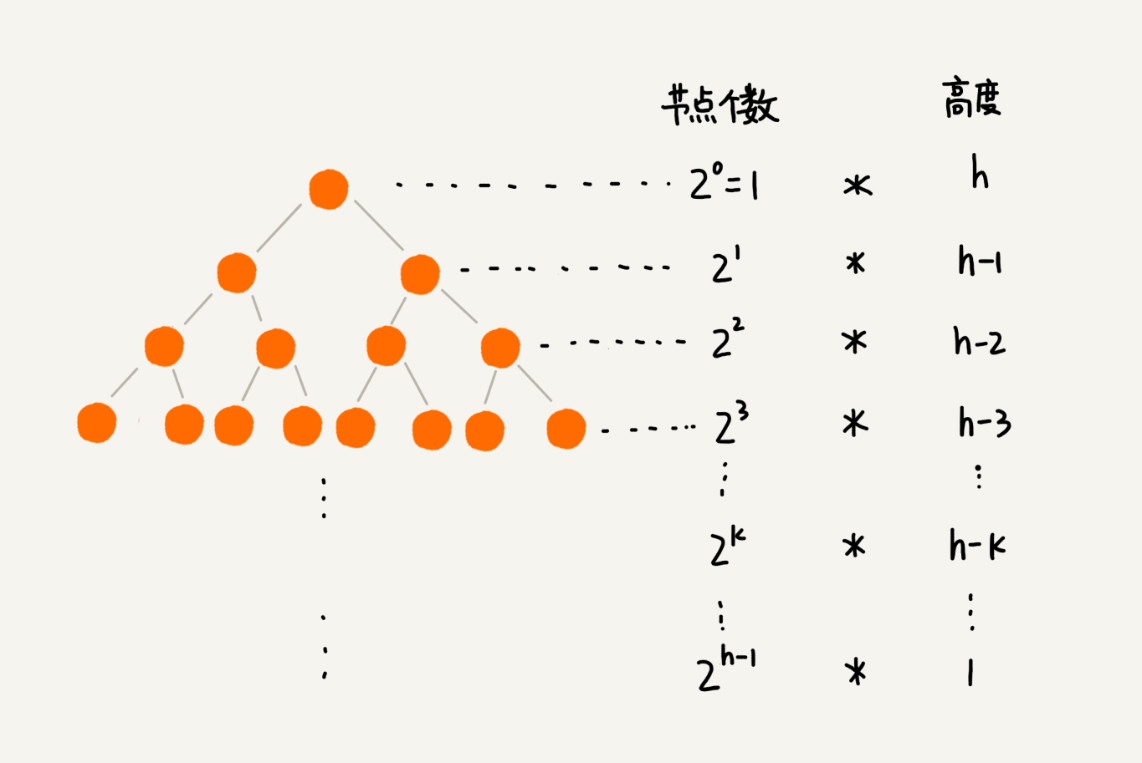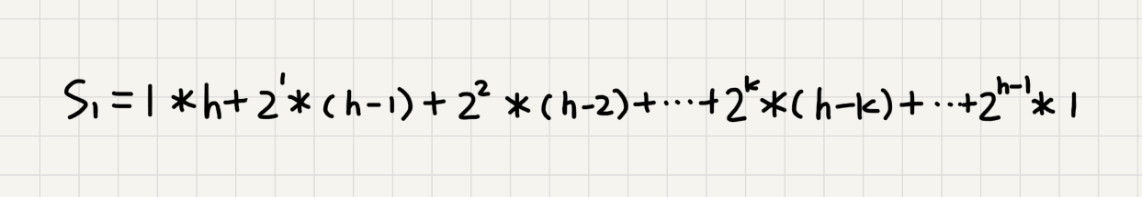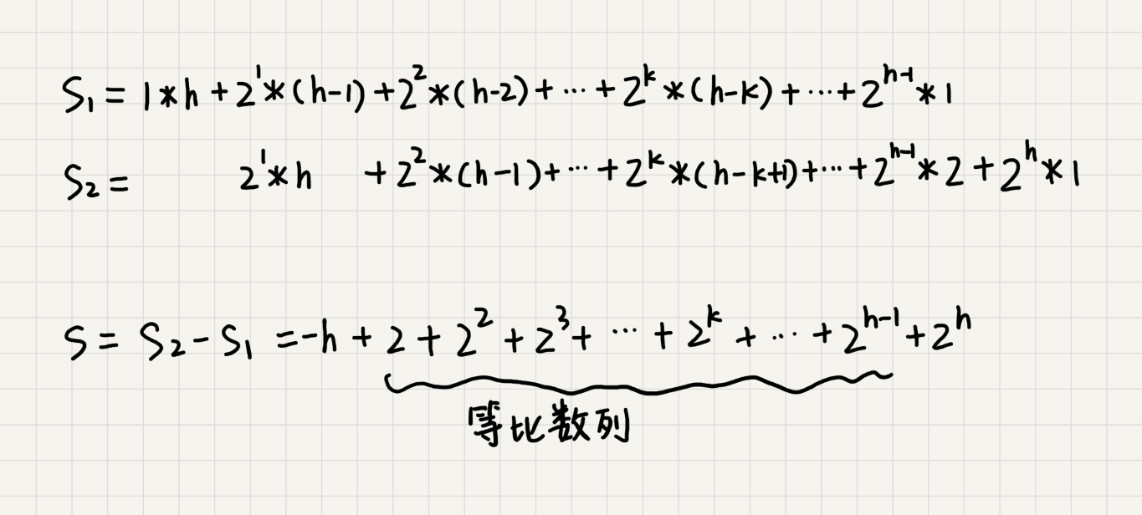$S$ 的中间部分是一个等比数列，所以最后可以用等比数列的求和公式来计算，最终的结果就是下面图中画的这个样子。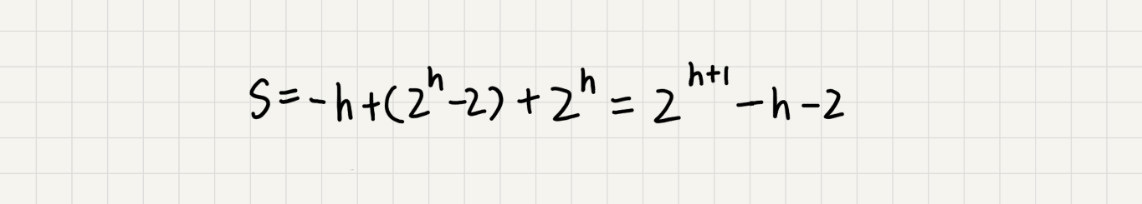2. 排序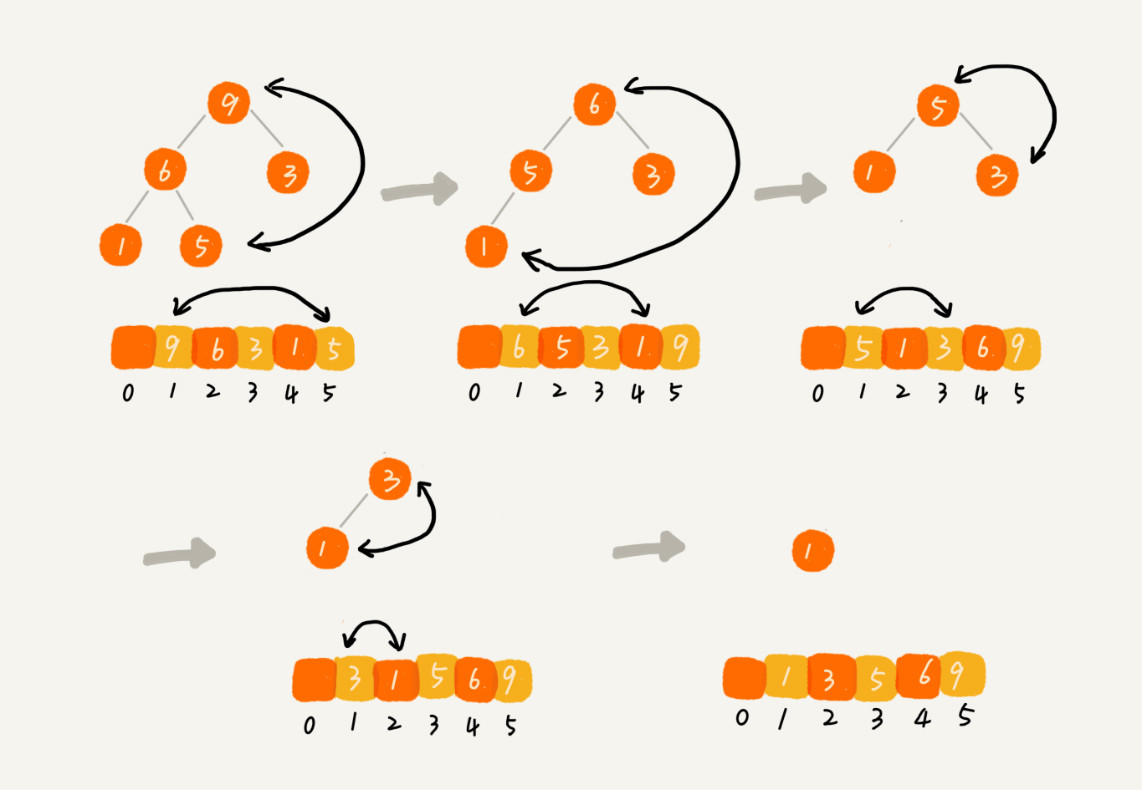// n 表示数据的个数，数组 a 中的数据从下标 1 到 n 的位置。
public static void sort(int[] a, int n) {
buildHeap(a, n);
int k = n;
while (k > 1) {
swap(a, 1, k);
--k;
heapify(a, k, 1);
}
}

## 思考

• 在实际开发中，为什么快速排序要比堆排序性能好？

主要有两方面的原因:

第一点，堆排序数据访问的方式没有快速排序友好。
对于快速排序来说，数据是顺序访问的。而对于堆排序来说，数据是跳着访问的。
比如，堆排序中，最重要的一个操作就是数据的堆化。比如下面这个例子，对堆顶节点进行堆化，会依次访问数组下标是 $1，2，4，8$ 的元素，而不是像快速排序那样，局部顺序访问，所以，这样对 CPU 缓存是不友好的。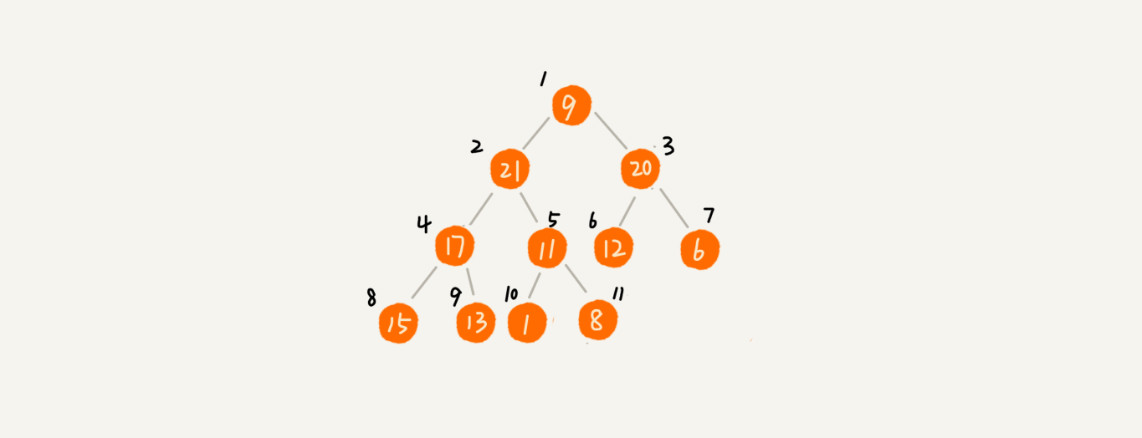第二点，对于同样的数据，在排序过程中，堆排序算法的数据交换次数要多于快速排序。
在写排序的时候，提过两个概念，有序度逆序度。对于基于比较的排序算法来说，整个排序过程就是由两个基本的操作组成的，比较和交换（或移动）。快速排序数据交换的次数不会比逆序度多。

但是堆排序的第一步是建堆，建堆的过程会打乱数据原有的相对先后顺序，导致原数据的有序度降低。比如，对于一组已经有序的数据来说，经过建堆之后，数据反而变得更无序了。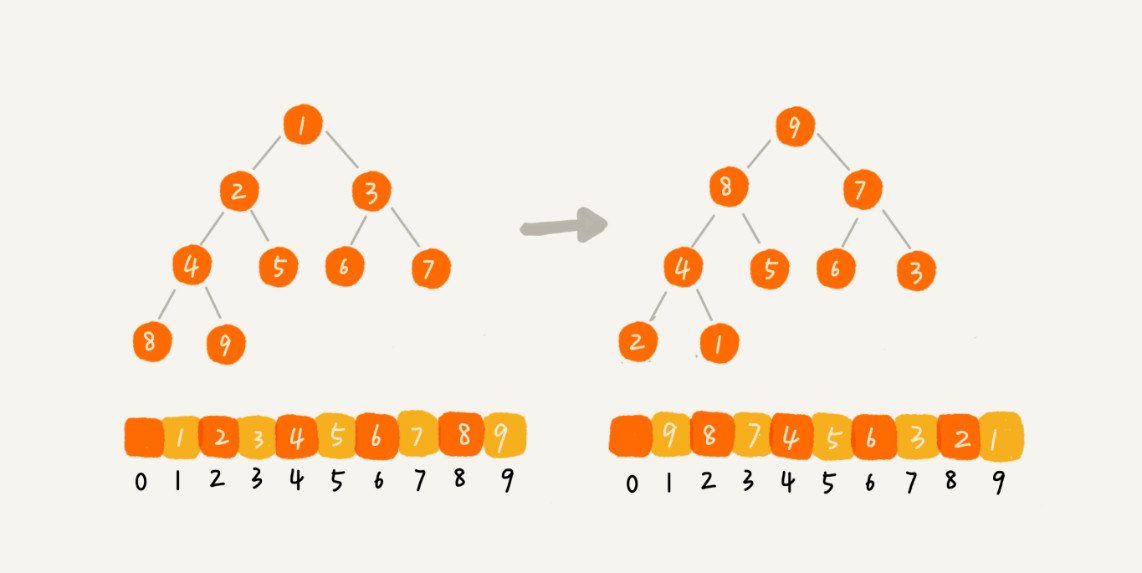对于第二点，可以自己做个试验看下。用一个记录交换次数的变量，在代码中，每次交换的时候，就对这个变量加一，排序完成之后，这个变量的值就是总的数据交换次数。这样就能很直观地理解刚刚说的，堆排序比快速排序交换次数多。

• 对于完全二叉树来说，下标从 $\frac n 2 +1$ 到 $n$ 的都是叶子节点，这个结论是怎么推导出来的呢？

使用数组存储表示完全二叉树时，从数组下标为$1$开始存储数据，数组下标为$i$的节点，左子节点为$2i$, 右子节点为$2i + 1$. 这个结论很重要（可以用数学归纳法证明)，将此结论记为『原理1』，以下证明会用到这个原理。

为什么，对于完全二叉树来说，下标从 $\frac n 2 +1$ 到 $n$ 的节点都是叶子节点？ 使用反证法证明即可：

如果下标为$\frac n 2 +1$的节点不是叶子节点，即它存在子节点，按照『原理1』，它的左子节点为：$2(\frac n 2 +1) = n + 2$，明显可以看出，这个数字已经大于$n + 1$，超出了实现完全二叉树所用数组的大小（数组下标从$1$开始记录数据，对于$n$个节点来说，数组大小是$n + 1$），左子节点都已经超出了数组容量，更何况右子节点。以此类推，很容易得出：下标大于$\frac n 2 +1$的节点肯定都是也叶子节点了，故而得出结论：对于完全二叉树来说，下标从 $\frac n 2 +1$ 到 $n$ 的节点都是叶子节点

• 堆的一种经典应用是堆排序。关于堆，你还能想到它的其他应用吗？

堆的应用除了堆排以外，还有如下一些应用：

• 从大数量级数据中筛选出$top n$ 条数据； 比如：从几十亿条订单日志中筛选出金额靠前的1000条数据
• 在一些场景中，会根据不同优先级来处理网络请求，此时也可以用到优先队列(用堆实现的数据结构)；比如：网络框架Volley就用了JavaPriorityBlockingQueue，当然它是线程安全的
• 可以用堆来实现多路归并，从而实现有序，leetcode上也有相关的一题：Merge K Sorted Lists